# Multiply Polynomials (With Examples): FOIL & Grid Methods

Melanie has a BS in physical science and is in grad school for analytics and modeling. She also runs a YouTube channel: The Curious Coder.

## What Is a Polynomial?

A polynomial can be made up of variables (such as x and y), constants (such as 3, 5, and 11), and exponents (such as the 2 in x2).

In 2x + 4, 4 is the constant and 2 is the coefficient of x.

Polynomials must contain addition, subtraction, or multiplication, but not division. They also cannot contain negative exponents.

The following example is a polynomial containing variables, constants, addition, multiplication, and a positive exponent:

3y2 + 2x + 5

Each segment in a polynomial that is separated by addition or subtraction is called a term (also known as a monomial.) The polynomial above has three terms.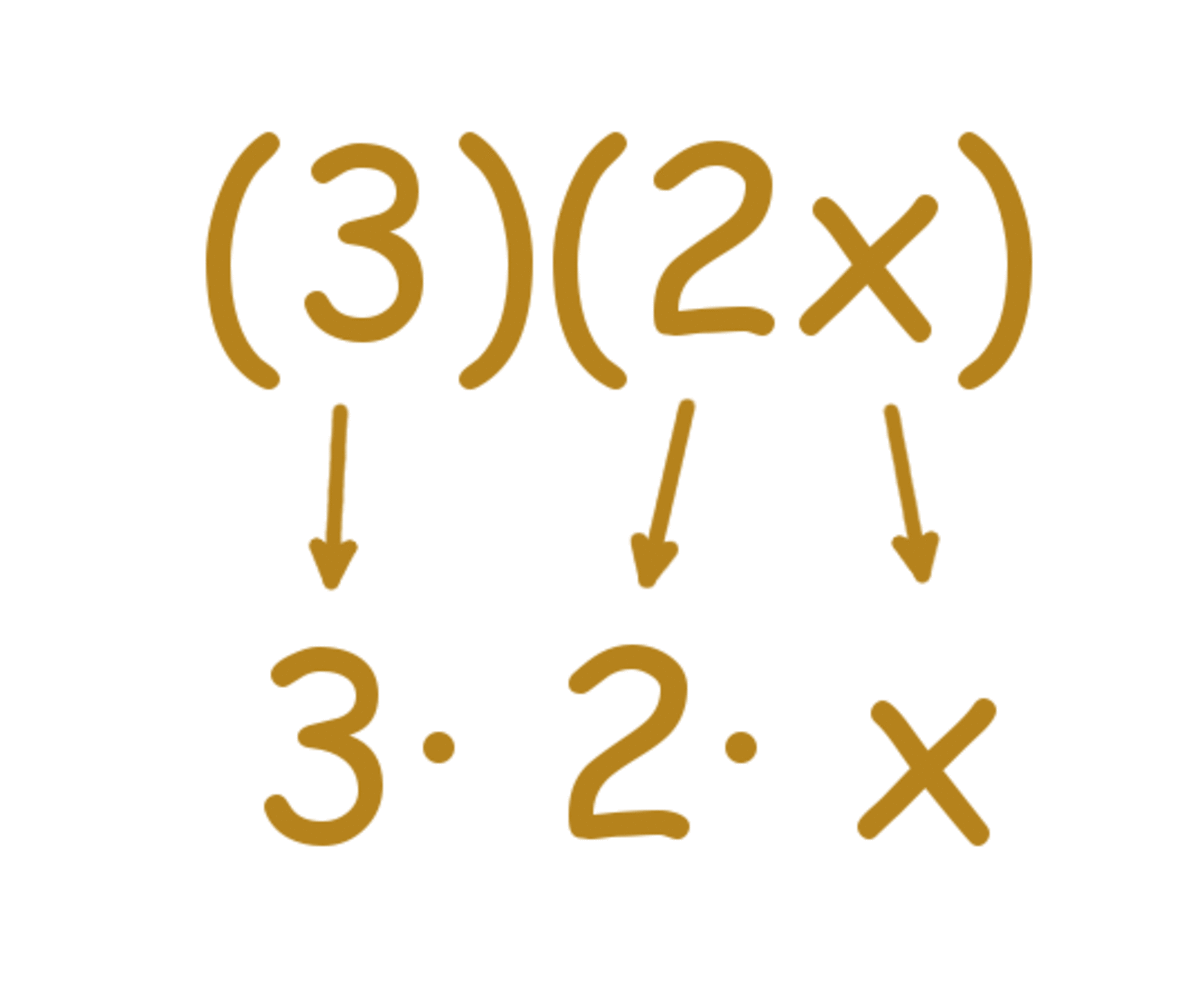(3)(2x) is like saying 3 times 2 times x.Melanie Shebel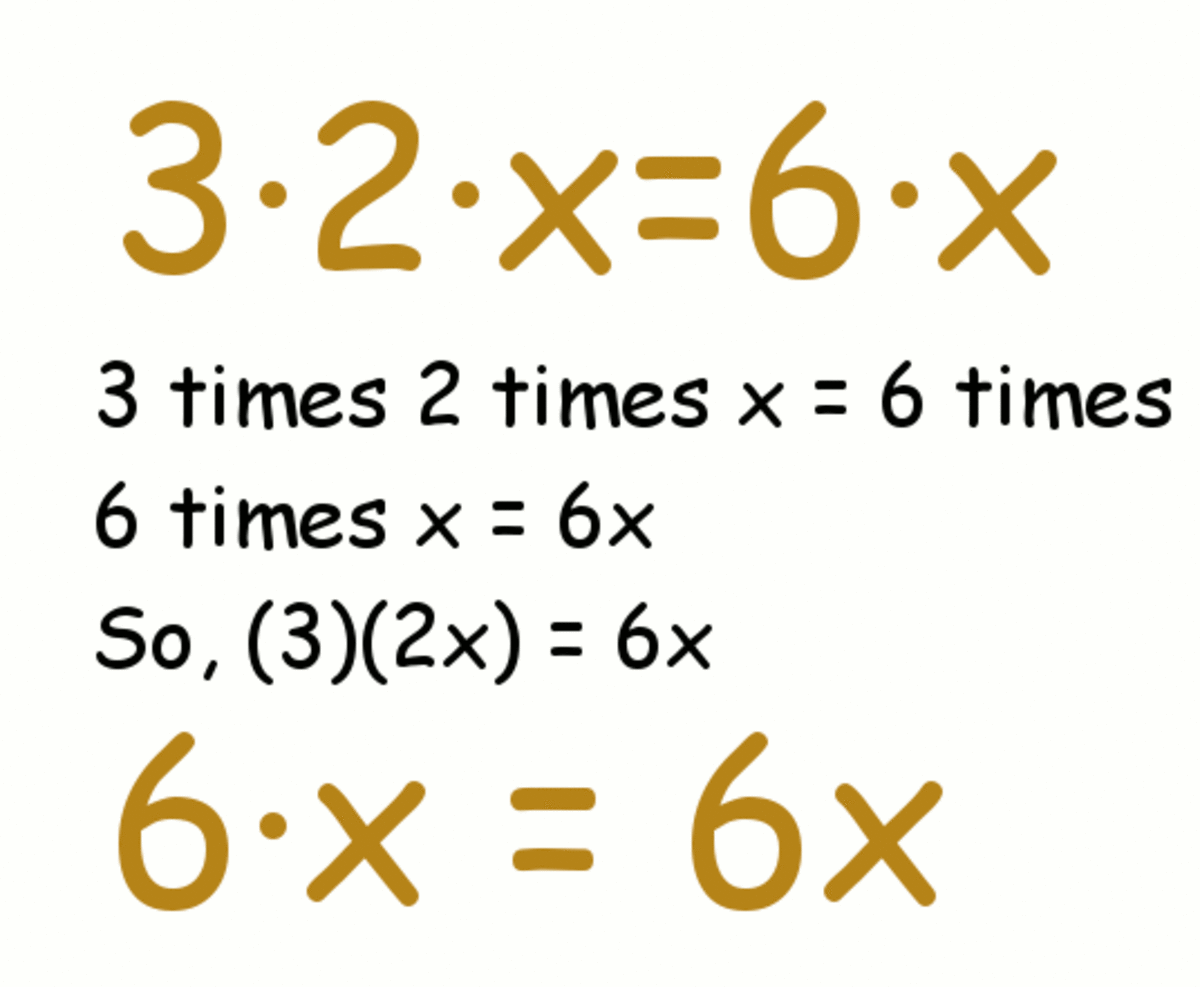Multiply three times two times x to get 6xMelanie Shebel

## Multiplying a Monomial Times a Monomial

Before jumping into multiplying polynomials, let's break it down into multiplying monomials. When you're multiplying polynomials, you'll be taking it just two terms at a time, so getting monomials down is important.

(3)(2x)

All you need to do here is break it down to 3 times 2 times x. You can get rid of the parenthesis and write it out like 3 · 2 · x. (Avoid using "x" to mean multiplication. It can get confusing with the letter x as a variable. Use · for multiplication instead!)

Because of the commutative property of multiplication, you can multiply the terms in any order, so let's solve this by going from left to right:

3 · 2 · x

3 times 2 is 6, so we're left with:

Scroll to Continue

## Read More From Owlcation

6 · x, which can be written as 6x.

## Practice: Multiplying Monomials

For each question, choose the best answer. The answer key is below.

1. (5)(4x) =
• 9x
• 20x
• 20
• 54x
2. (7)(x)
• 7x
• x
• 7
• 6
3. (1)(2x)
• 12x
• 12
• x
• 2x

1. 20x
2. 7x
3. 2x

## Quick Refresher on Multiplying Exponents

When adding exponents, you add the coefficients.

2x + 3x = 5x.

x + x = 2x

So what do you do when multiplying exponents?

x · x = ?

When multiplying like variables with exponents, you just add the exponents.

(x2)(x3) = x5

This is the same as saying x · x · x · x · x

(2x)(5xy) = 10x2y

This is the same as saying 2 · x · 5 · x · y or 2 · 5 · x · x · y

Remember that x = x1. If no exponent is written, it's assumed that it's to the first power. This is because any number is equal to itself to the first power.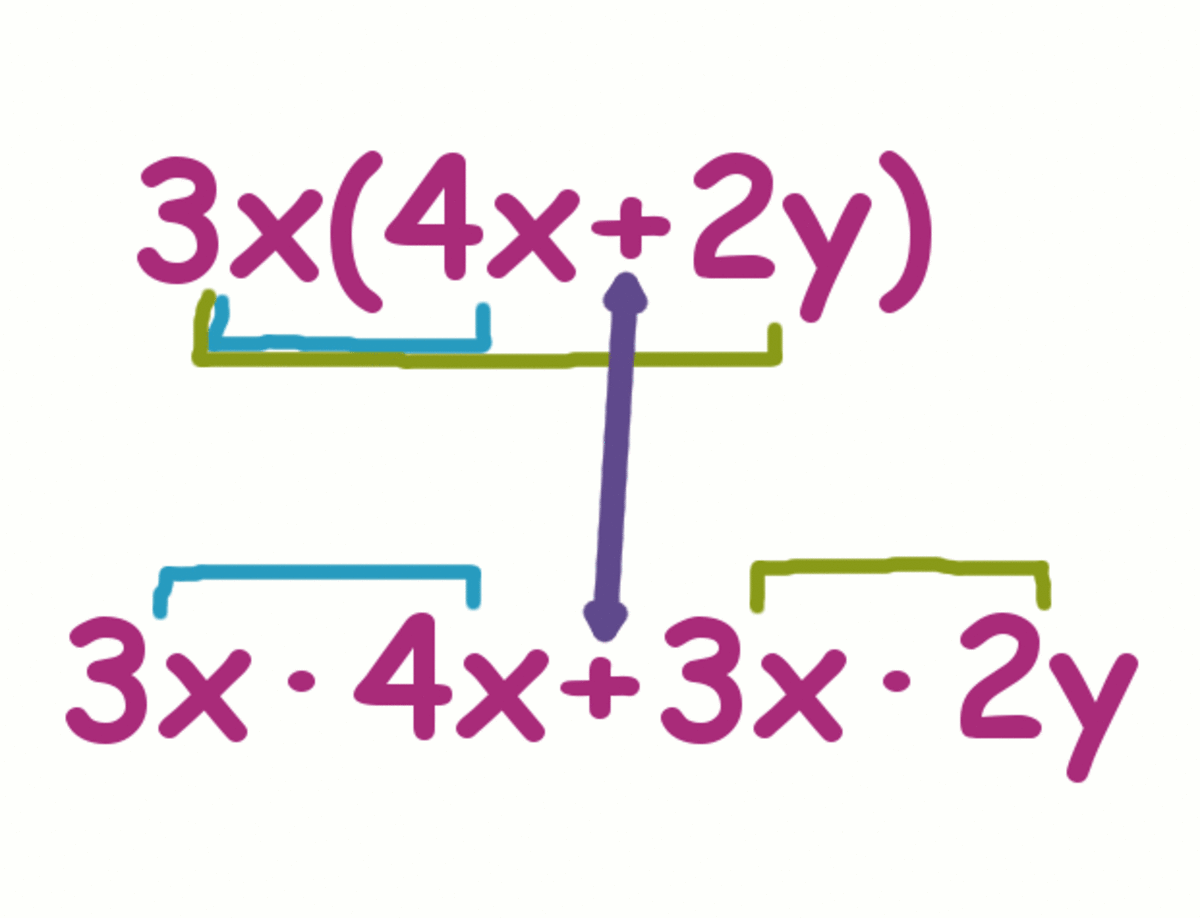Multiplying 1 term by 2 terms: Write down 3x times 4x + 3x times 2x.Melanie Shebel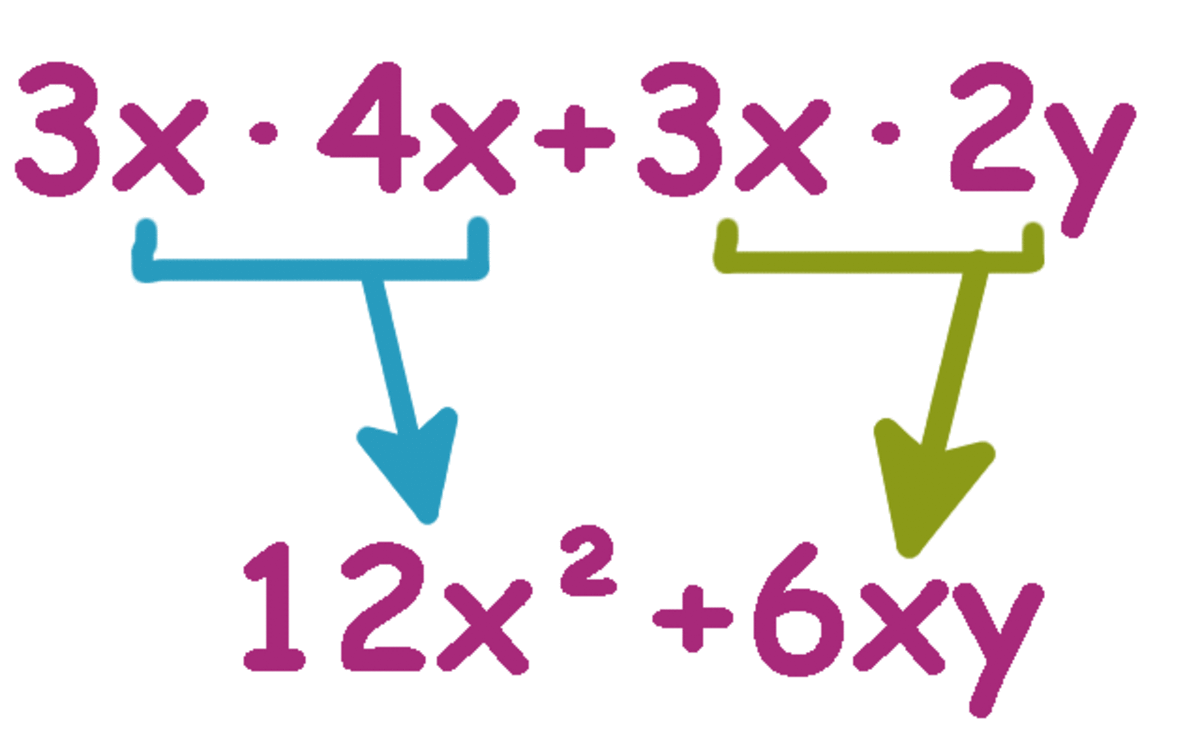3x times 4x is 12x² and 3x times 2y is 6xy.Melanie Shebel

## Multiplying 1 Term by 2 Terms

When multiplying one term by two terms, you have to distribute them into the parenthesis.

Sample problem:

3x(4x+2y)

Step 1: Multiply 3x times 4x. Write down the product.

Step 2: Write down a plus sign, since there's addition in the parenthesis and the product of 3x and 2y is positive.

Step 3: Multiply 3x times 2y. Write down the product.

You should have 12x2 + 6xy written down. Since there are no like terms to add together, you're done.

If you're dealing with negative numbers or subtraction, you have to watch the signs.

For example, if the problem is -3x(4x+2y), you'll have to multiply negative 3x times everything in the parenthesis. Since the product of -3x and 4x is negative, you would have -12x2. Then, it would be -6xy since the product of -3x and 2y are negative (if the plus sign throws you off, you can write it as 12x2 + -6xy.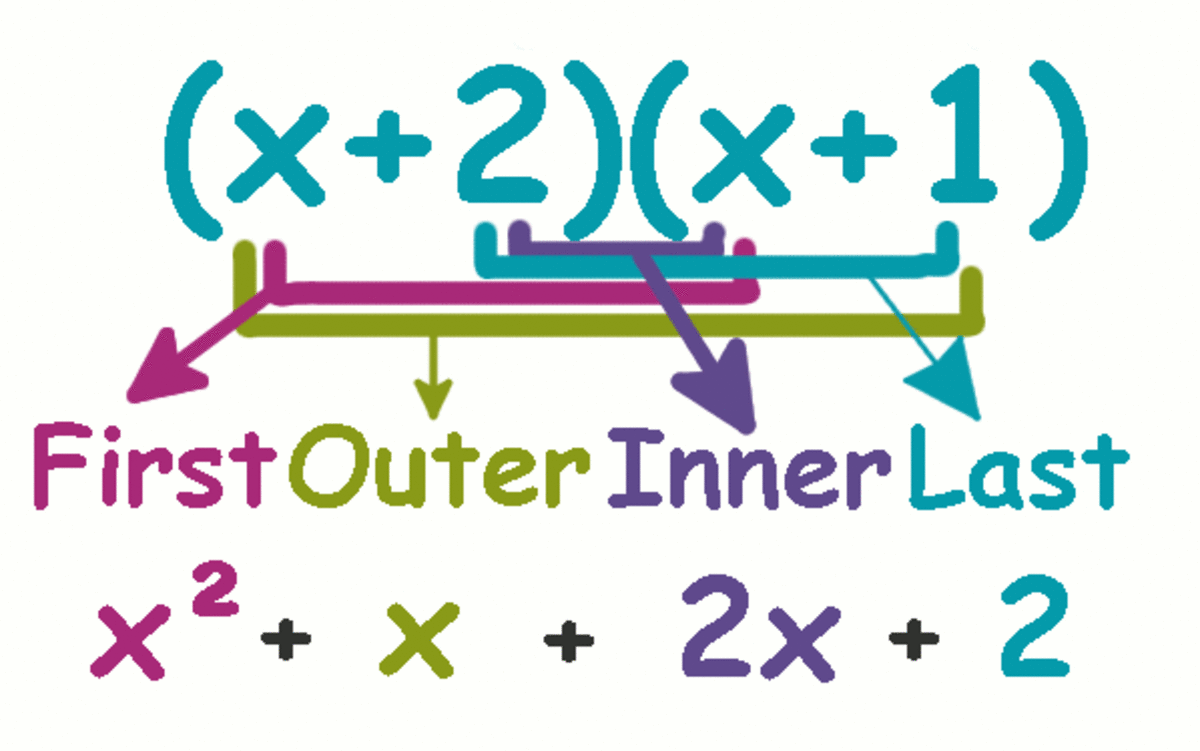The FOIL method: Multiply the first terms, the outer, inner, and then finally the last terms. Combine like terms and voila!Melanie Shebel

## Multiplying Binomials Using the FOIL Method

A polynomial with just two terms is called a binomial. When you're multiplying two binomials together, you can use an easy-to-remember method called FOIL.

FOIL stands for First, Outer, Inner, Last.

Sample problem:

(x+2) (x+1)

Step 1: Multiply the first terms in each binomial. The first terms here are the x from (x+2) and the x from (x+1). Write down the product. (The product of x times x is x2.)

Step 2: Multiply the outer terms in each of the two binomials. The outer terms here are the x from (x+2) and the 1 from (x+1). Write down the product. (The product of x times 1 is 1x, or x.)

Step 3: Multiply the inner terms in the two binomials. The inner terms here are the 2 from (x+2) and the x from (x+1). Write down the product. (The product of 2 times x is 2x.)

Step 4: Multiply the last terms in each of the two binomials. The last terms here are the 2 from (x+2) and the 1 from (x+1). Write down the product. (The product of 1 times 2 is 2.)

You should have: x2 + x + 2x + 2

Step 5: Combine like terms. There is nothing here with an x2 attached to it, so x2 stays as is, x and 2x can be combined to equal 3x, and 2 stays as is because there are no other constants.

Your final answer is: x2 + 3x + 2

## Practice: Multiplying Polynomials

For each question, choose the best answer. The answer key is below.

1. (x+2)(x+6)
• x²+8x+12
• x+8
• x²+2x+6
• 8x
2. (x-3)(x+4)
• x²-x+12
• x
• x²+12x+1
• x²+x-12
3. (x+7)(x²+2x+1)
• 7x²+3x+8
• x³+9x²+15x+7
• 71x³+9x²+x+1
• None of the above

1. x²+8x+12
2. x²+x-12
3. x³+9x²+15x+7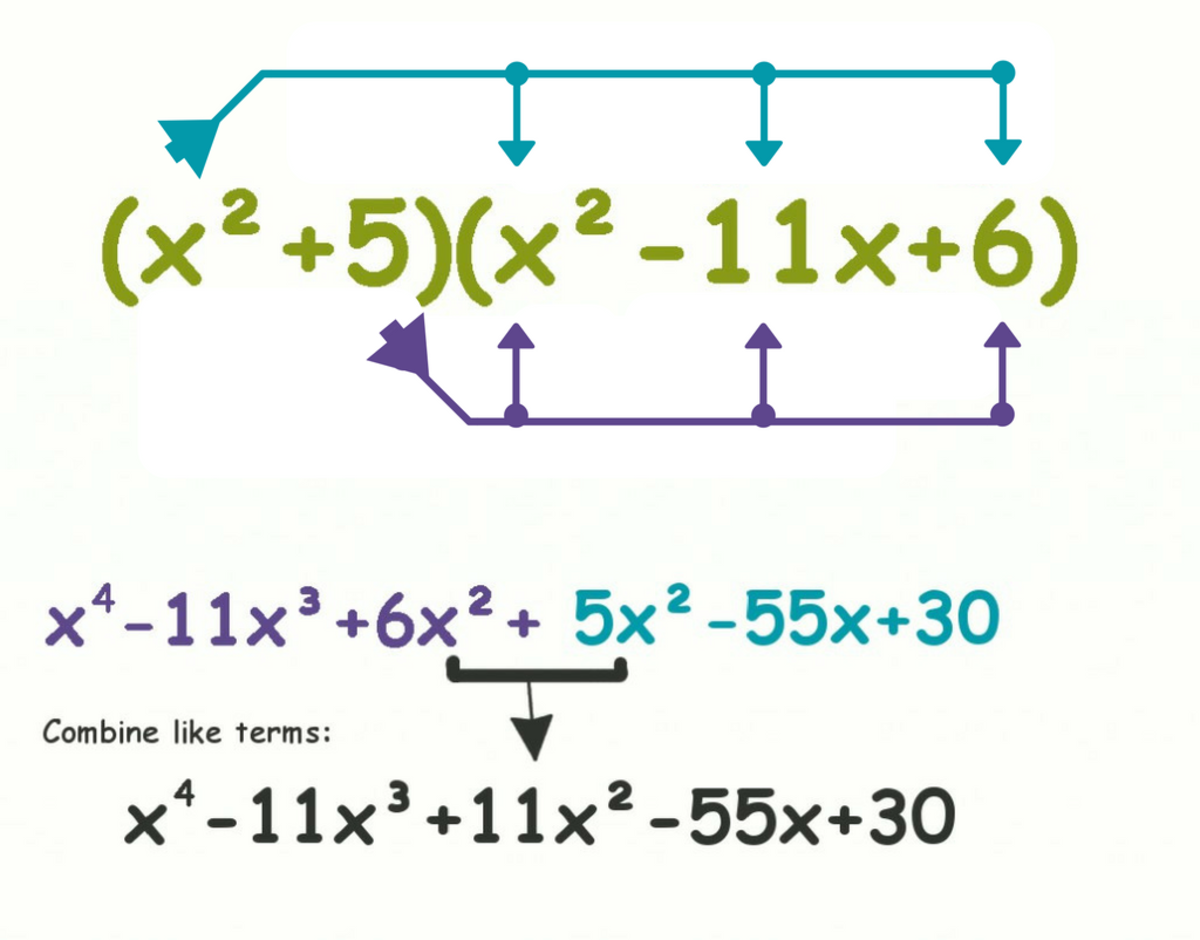Distributing terms without FOIL: Distribute each term in one polynomial to each term in the other polynomial.

## Distributing Polynomials (Without FOIL)

When you're dealing with the multiplication of two polynomials, order them so that the polynomial with fewer terms is to the left. If the polynomials have an equal number of terms, you can leave it as is.

For example, if your problem is: (x2-11x+6)(x2+5)
Rearrange it so it looks like: (x2+5)(x2-11x+6)

Step 1: Multiply the first term in the polynomial on the left by each term in the polynomial on the right. For the problem above, you would multiply x2 by each x2,-11x, and 6.
You should have x4-11x3+6x2.

Step 2: Multiply the next term in the polynomial on the left by each term in the polynomial on the right. For the problem above, you would multiply 5 by each x2,-11x, and 6.
Now, you should have x4-11x3+6x2+5x2-55x+30.

Step 3: Multiply the next term in the polynomial on the left by each term in the polynomial on the right. Since there are no more terms in the left polynomial in our example, you can go ahead and skip to step 4.

Step 4: Combine like terms.
x4-11x3+6x2+5x2-55x+30 = x4-11x3+11x2+-55x+30

## Multiplying Using a Grid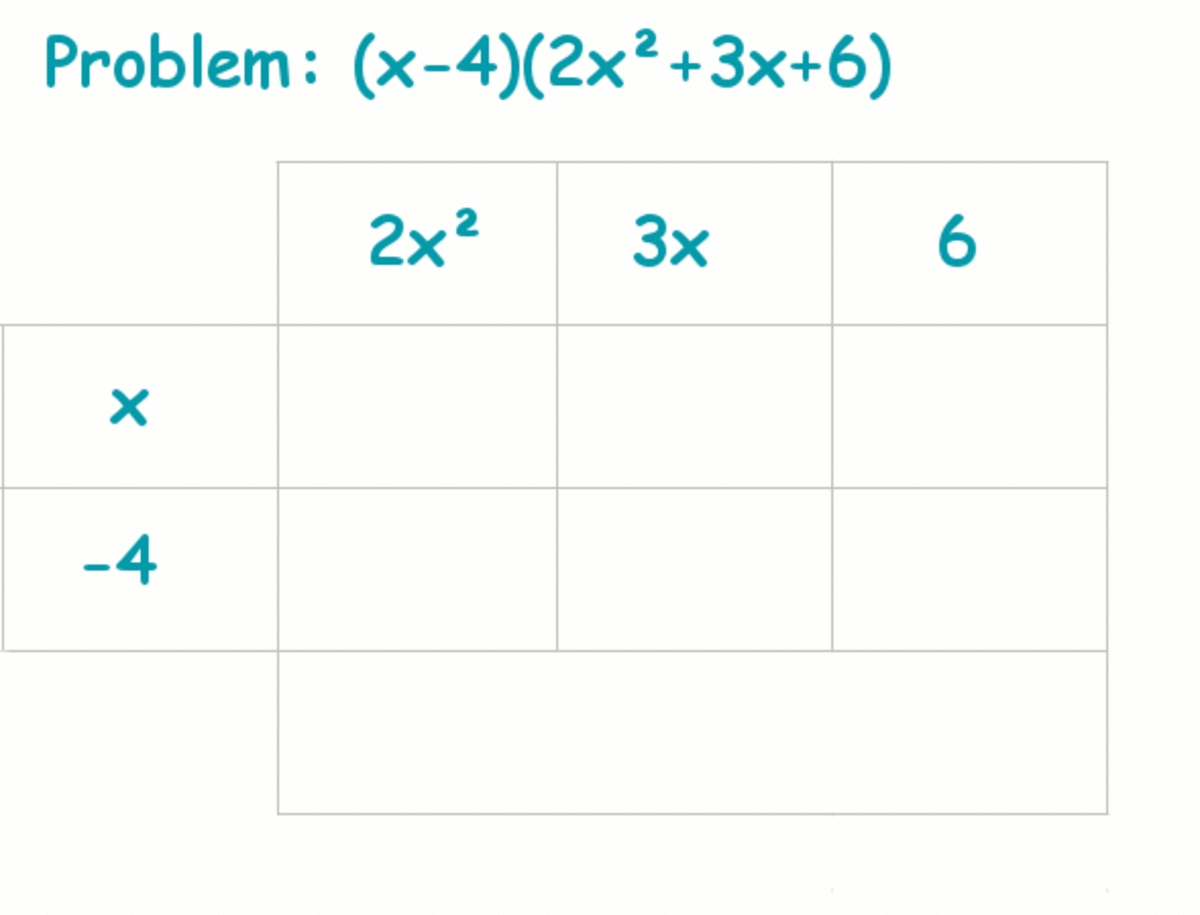Start off with a grid containing the terms one one polynomial across the top and the terms of the other down the side.Melanie Shebel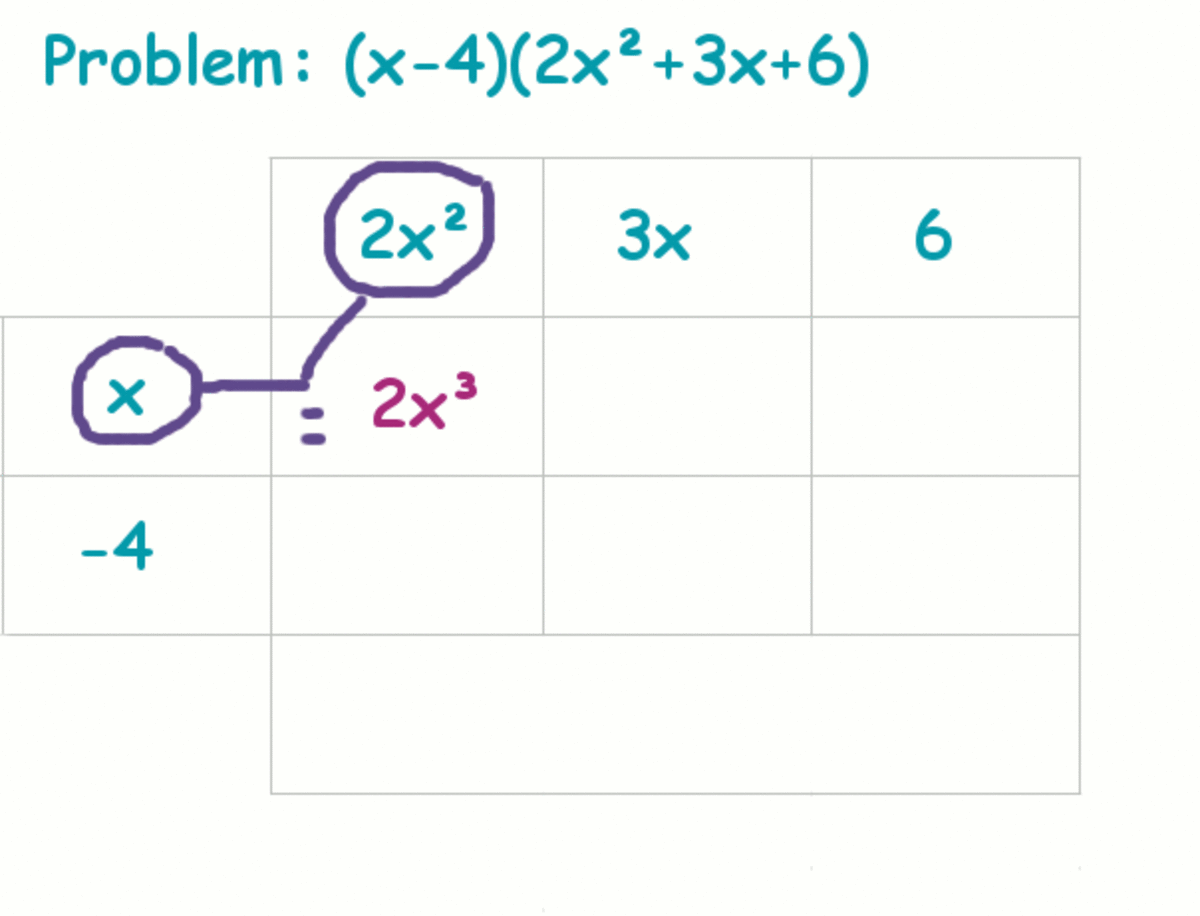Multiply term in the first row by the term in the first column. Write down the product.Melanie Shebel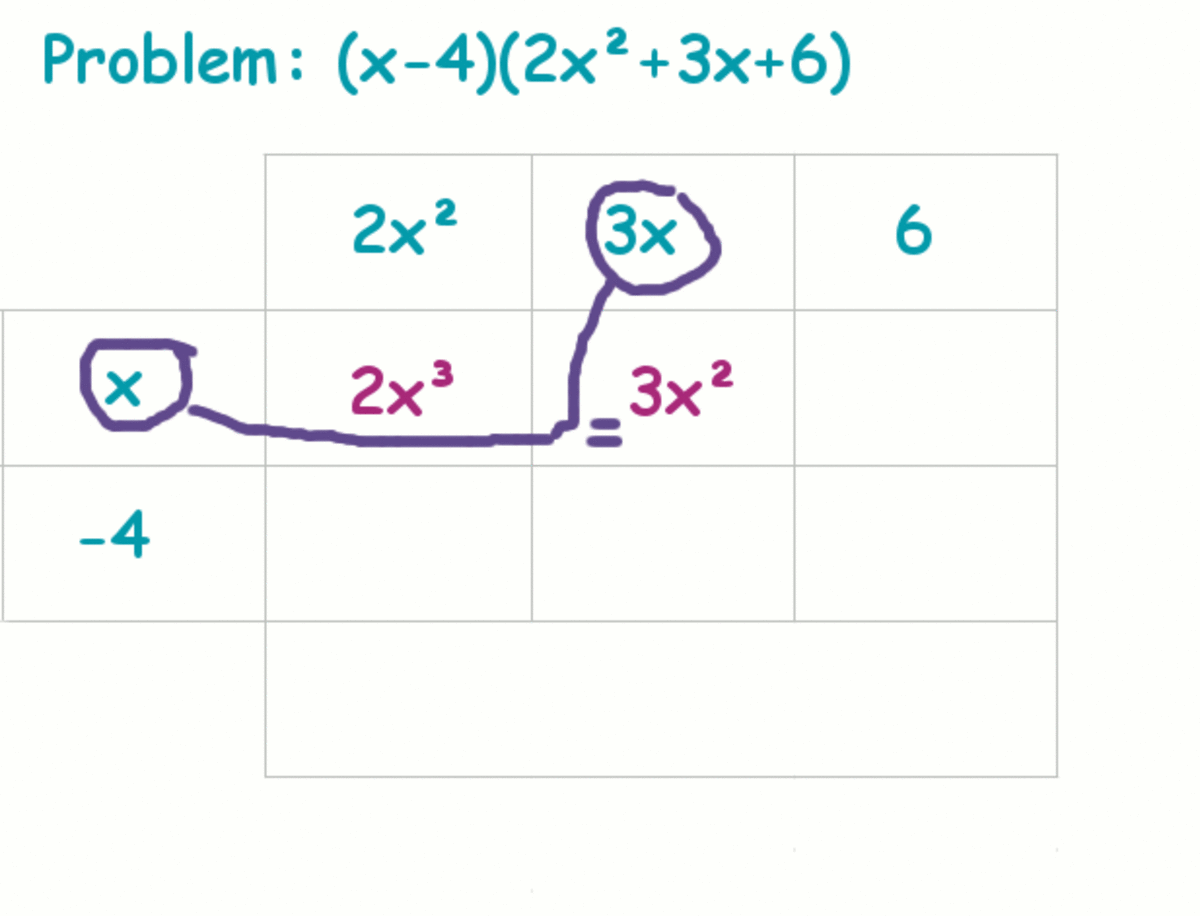Continue by filling in the next box with the product of the terms in the corresponding column and row.Melanie Shebel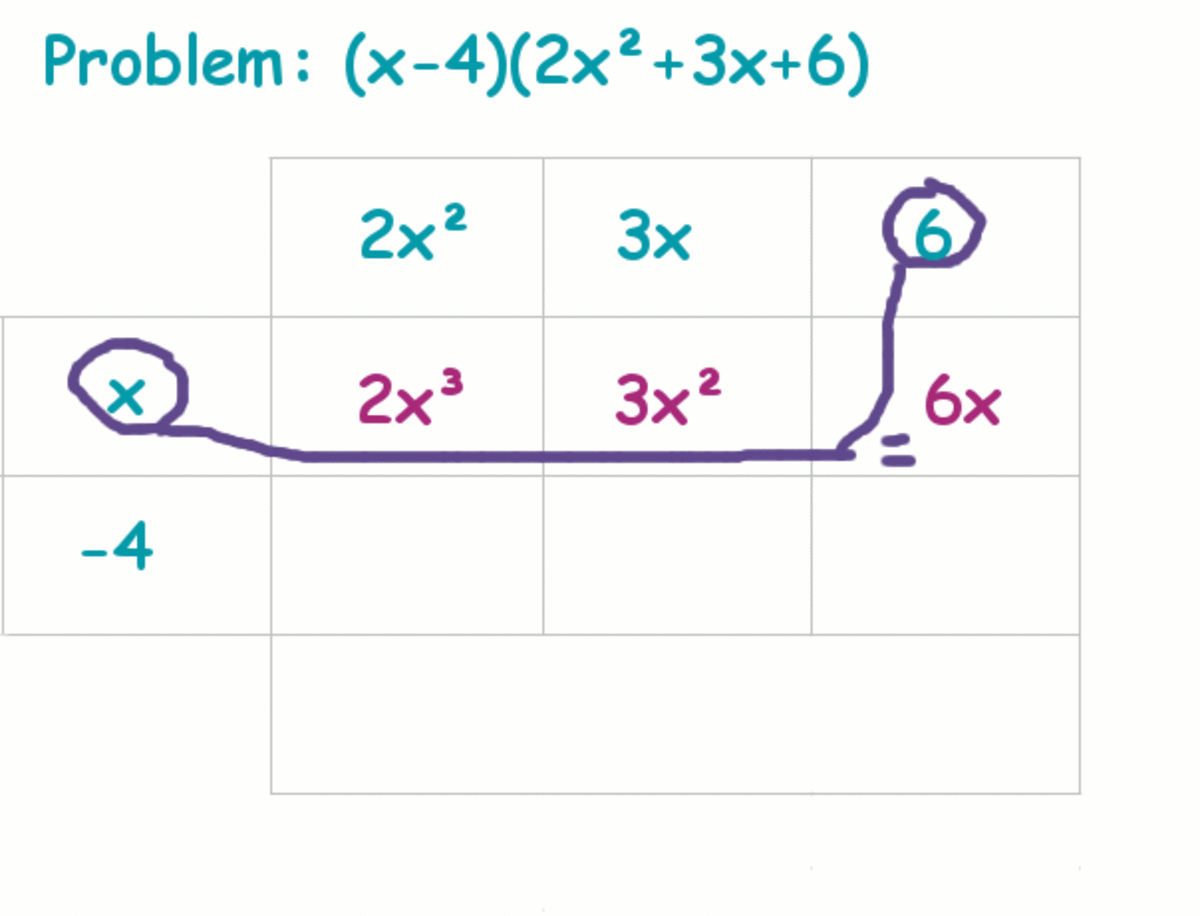Fill in each box in the grid.Melanie Shebel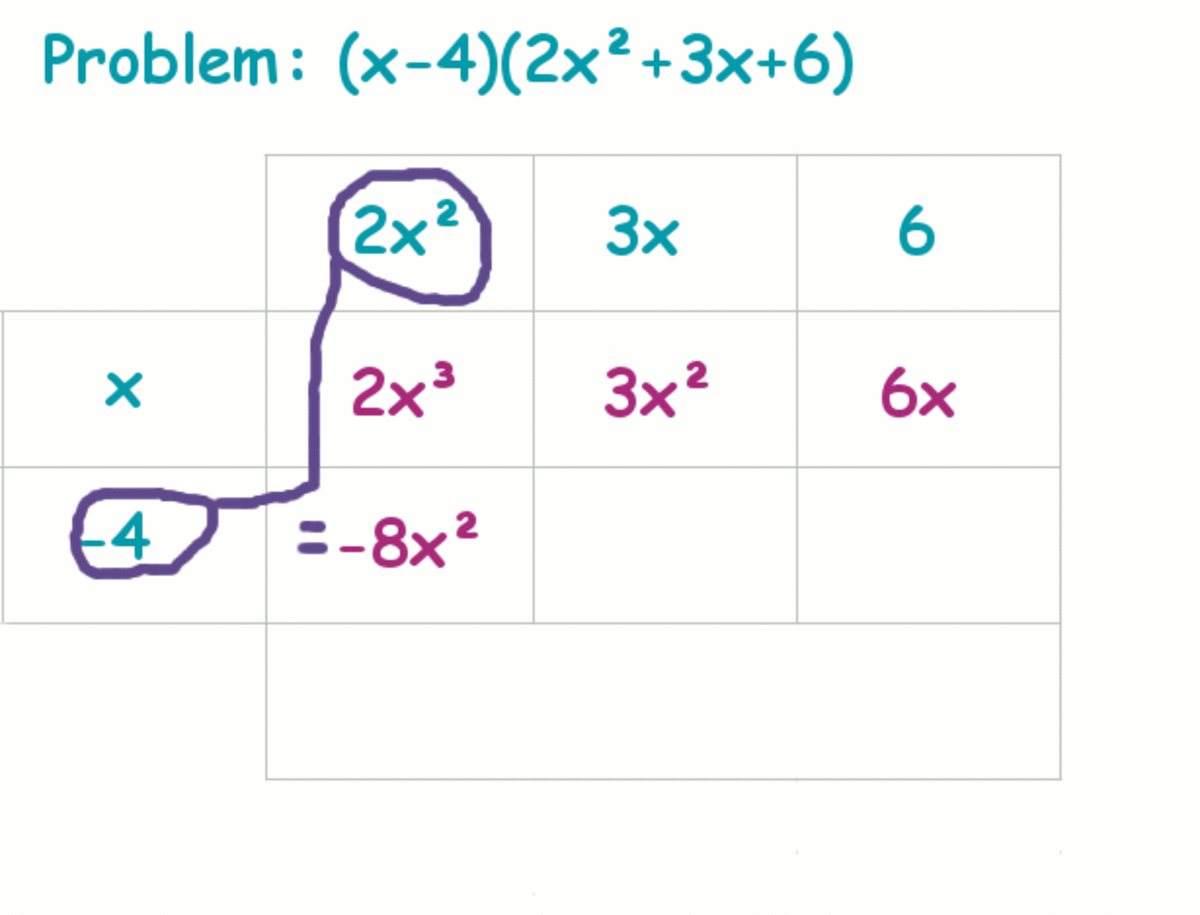Here we're starting on the next row.Melanie Shebel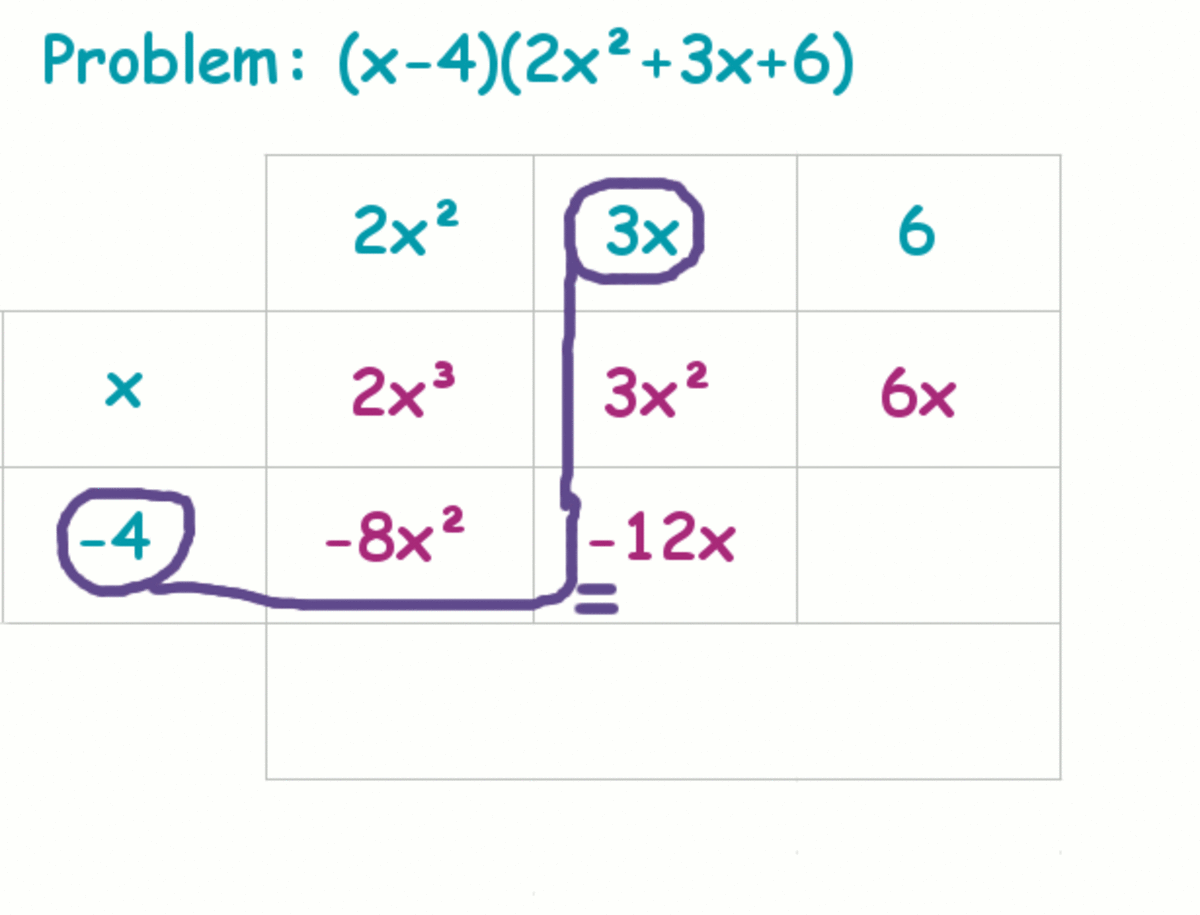Continue finding the products of termsMelanie Shebel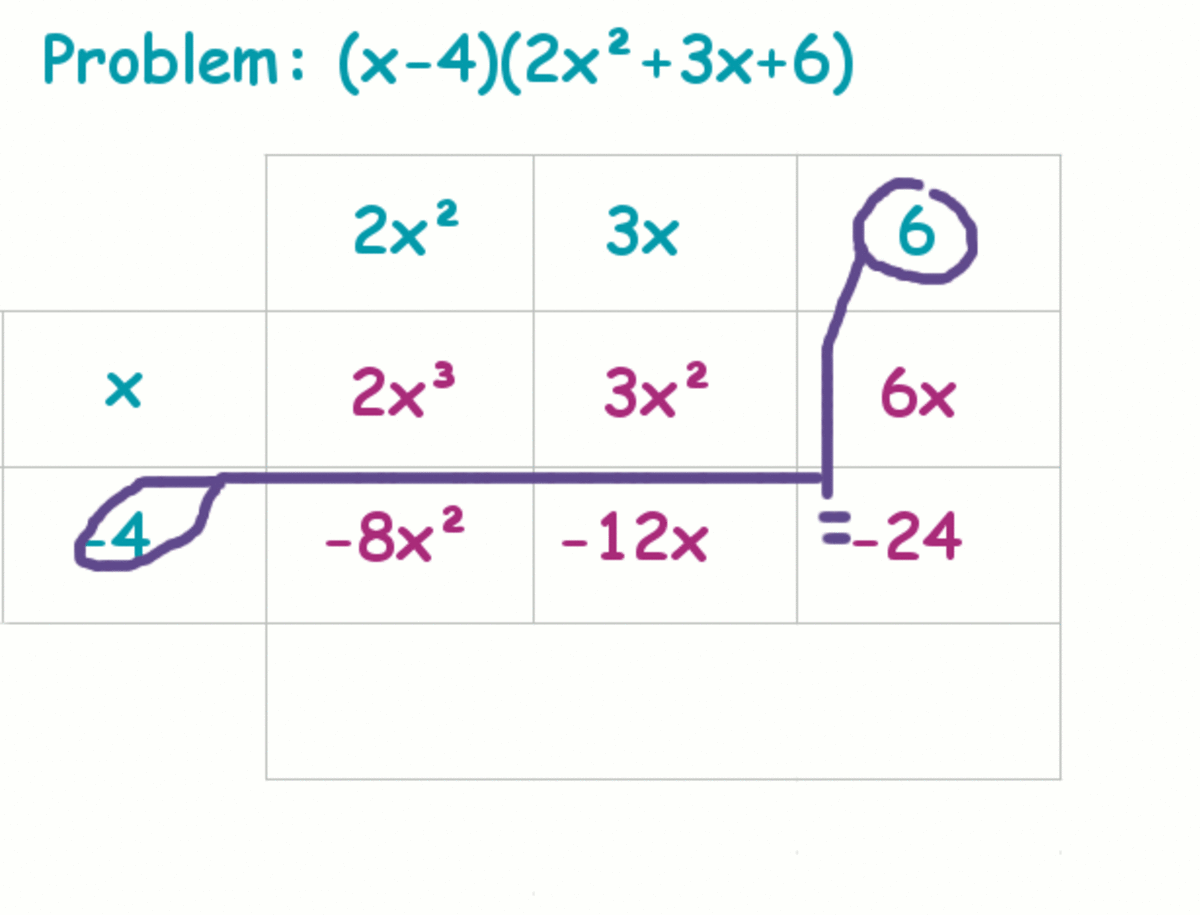Yay! We've got all the products we need! The hard part is done!Melanie Shebel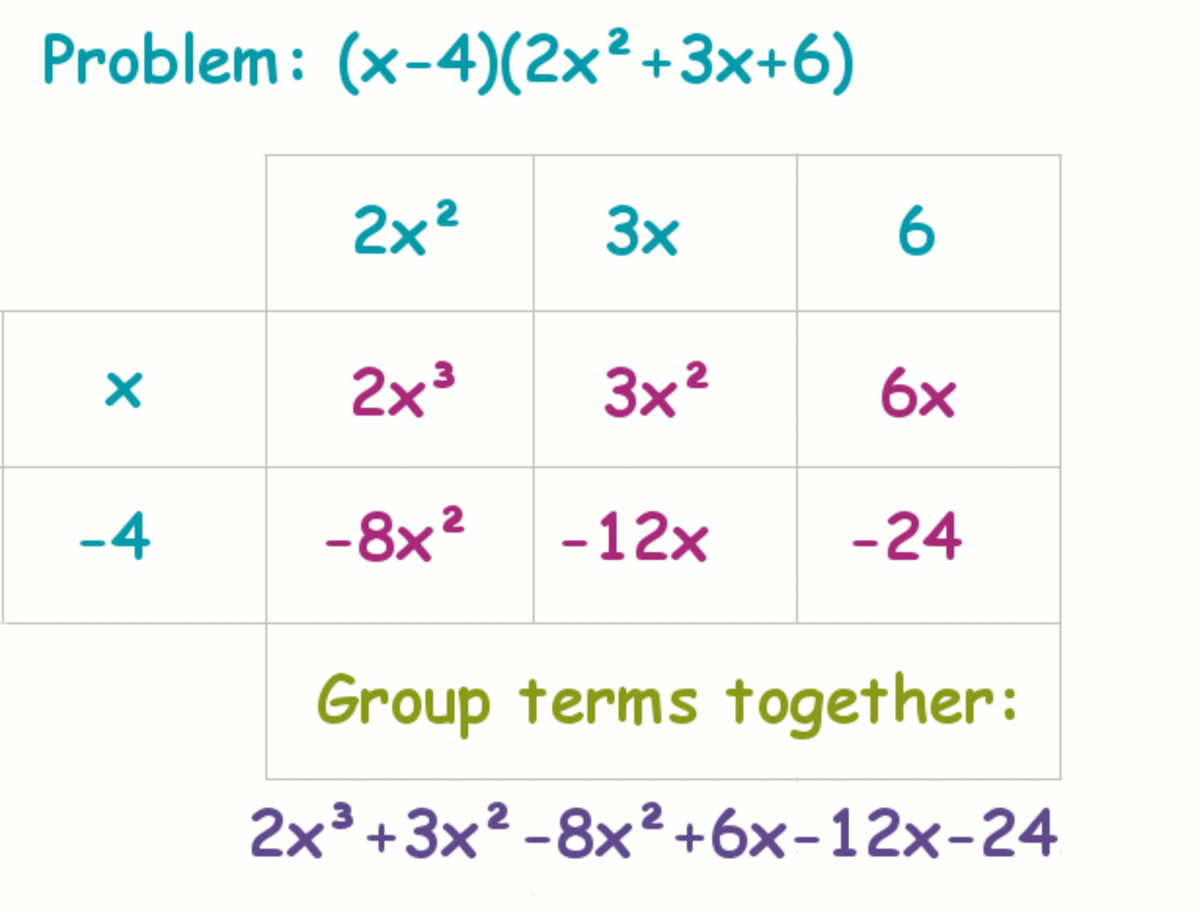Group together like terms (this will make it easier to find all the sums and differences.)Melanie Shebel

## Using the Grid Method

One of the biggest drawbacks of using the FOIL method is that it can only be used for multiplying two binomials. Using the distribution method can get really messy, so it's easy to forget to multiply some terms.

The best way to multiply polynomials is the grid method. This is actually just like the distribution method except everything goes right into a handy grid making it almost impossible to lose terms. Another thing that's nice about the grid method is that you can use it to multiply any type of polynomials whether they're binomials or have twenty terms!

Start off by making a grid. Put each term in one of the polynomials across the top and the terms of the other polynomial down the left side. In each box in the grid, fill in the product of the term for the row times the term for the column. Combine like terms and you're done!

Leave a comment below if you're still struggling. I want to create the perfect guide to multiplying polynomials and if there is something you don't quite understand.

## Questions & Answers

Question: Do we need to arrange polynomials alphabetically?

Answer: While this isn't a requirement, arranging polynomials alphabetically is a really good practice because it helps you notice patterns (especially when combining like terms) as well as make fewer mistakes. Since it's so handy to have polynomials arranged alphabetically, I'm tempted to just say, "Yes, you need to arrange them alphabetically."

© 2012 Melanie Palen

Hessa Humaid on March 28, 2020:

I am going to have a big test for this, and this has helped me a lot, thank you :)

Atyq on October 24, 2012:

I am learning it at school, but I didn't how to multiply these polynomials.

Thanks for your tips...

Katina Davenport on April 30, 2012:

I remember these well from high school and college. Great tutorial for who ever needs to learn polynomials.

Katina

emilybee on April 30, 2012:

I use to love FOIL, it was basically the only thing related to polynomials that I understood. Math was not my strong point ;) Great hub, and very helpful for many!

Jessee R from Gurgaon, India on April 30, 2012:

Great hub! Great basics to learn and the Foil method is very well explained here!

David Warren from Nevada and Puerto Vallarta on April 02, 2012:

This is a phenomenal hub! I am taking an online course at M.I.T. and my math skills are a bit rusty as I'm not so young anymore,lol. Anyway thank you for sharing this, great job! Voted up and awesome!

freemarketingnow from California on March 15, 2012:

Now, the next question is: What is the real life application of multiplying polynomials?

India Arnold from Northern, California on March 14, 2012:

Easy to follow, simply explained, and clearly drawn examples made the learning process a positive experience. (Go figure, you made math a 'positive' experience! he-he...)

This really is a helpful teaching guide for learning how to multiply polynomials. Great hub!

HubHugs~

Brittany Kennedy from Kailua-Kona, Hawaii on March 12, 2012:

Ditto to what Simone said! This was so easy-to-read and clear; I am going to send it to my brother who takes algebra! What a wonderful hub! Great work, voted up, useful, etc!

Simone Haruko Smith from San Francisco on March 12, 2012:

MELBEL!!! WHERE WERE YOU WHEN I HAD TO LEARN THIS STUFF FOR THE FIRST TIME!?!!?!?!1111

You've illustrated the process splendidly, and the diagram things you've provided are super helpful. Heck... I think I almost... LIKE multiplying polynomials now!

Melanie Palen (author) from Midwest, USA on March 12, 2012:

Middle school math was not fun for me! I think it's awesome that you'll be helping your daughter in math. Algebra was way over my parents' heads, so I was on my own! :P

Order of opps is PEMDAS: Parenthesis, Exponents, Multiplication/Division, and Addition/Subtraction. A way to remember this, which you've probably heard before: "Please Excuse My Dear Aunt Sally." I hope that helps!

Kelly Umphenour from St. Louis, MO on March 11, 2012:

Hey Melbel! Cool - I gotta keep up - my middle daughter is now in middle school....algebra has begun! Ohhh I don't even remember the correct order of operations now! But - I know exactly where I'm going when I hear her utter polynomials! Thanks - in advance:)

Marisa Hammond Olivares from Texas on March 11, 2012:

Wow Melanie! I love the graphics and colors you used! The grid graphics and format are a great addition to this awesome hub. I did take the quiz, but I think I'll keep my score to myself. LOL, but seriously - you really did lay this out nicely and explained the steps and concept clearly.

Nice job and a great lesson - thanks!

Melanie Palen (author) from Midwest, USA on March 11, 2012:

Agreed. I'll still FOIL for binomials, but anything larger will be distributed or put into the grid (since distributing can get messy.)

freemarketingnow from California on March 11, 2012:

It's good. I like the checks for understanding with the quizzes. I prefer it to use a grid format. FOIL is so old-school (from the days we did it in school). It only works for binomials, but it doesn't work with others (like trinomial times trinomial)

Xavier Nathan from Isle of Man on March 11, 2012:

You have done a great job explaining this topic and the colours really do make a difference. Now I look forward to reading your next hub on factorizing polynomials! :)

Cloverleaf from Calgary, AB, Canada on March 11, 2012:

An excellent refresher! You've taken me back to my good old days at school. I really enjoyed how you used the examples and illustrations throughout, very well explained. Voted up!

Melanie Palen (author) from Midwest, USA on March 11, 2012:

Wow! Thank you! I was actually really debating which colors to use, but decided to go with something that looked kind of hubpages-y. I'm glad you liked the color-scheme. :)

Patty Inglish MS from USA and Asgardia, the First Space Nation on March 10, 2012:

I like the use of colors very much. Rated Up and Awesome and more.

anuramkumar from Chennai, India on March 10, 2012:

Found it very useful. You have explained the concept in very simple terms.